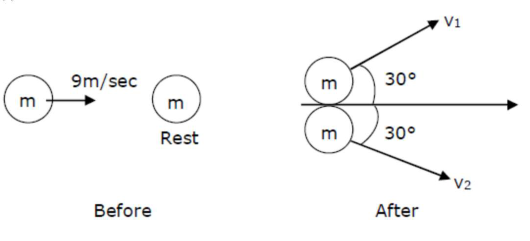# A ball with a speedQuestion:

A ball with a speed of $9 \mathrm{~m} / \mathrm{s}$ collides with another identical ball at rest. After the collision, the direction of each ball makes an angle of $30^{\circ}$ with the original direction. The ratio of velocities of the balls after collision is $\mathrm{x}: \mathrm{y}$, where $\mathrm{x}$ is

Solution:Momentum is conserved just before and just after the collision in both $x-y$ direction.

In $y$-direction

$\mathrm{p}_{\mathrm{i}}=0$

$P_{f}=m \times \frac{1}{2} v_{1}-m \times \frac{1}{2} v_{2}$

$\mathrm{p}_{\mathrm{i}}=\mathrm{p}_{\mathrm{f}}$, so

$=\frac{m v_{1}}{2}-\frac{m v_{2}}{2}=0$

$\Rightarrow \frac{m v_{1}}{2}=\frac{m v_{2}}{2} \Rightarrow v_{1}=v_{2}$

$\frac{\mathrm{v}_{1}}{\mathrm{v}_{2}}=1$